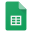# Calculating linkage disequilibrium (LD) from Pool-seq data

[ 2019-12-06]

• Pool sequencing data does not contain individual genotype data and hence the linear combinations of alleles per genotype cannot be known. In other words haplotype information is lost.
• What we know are allele frequencies per pool
• We have linear allele combination information per read which is limited by the sequencing technology used, i.e. for the hugely popular and currently state-of-the-art short-read fluorescence-based sequencing technology by Illumina, this is limited to 150bp tp 300bp, and an impressive ~2Mbp using nanopore sequencing.
• Considering short-read sequencing, should we look for overlapping sequences (which can be very rare if they exist at all) to somehow piece-together a longer sequence. This approach will not work for deliberately sparse genome coverage approches such as genotyping-by-sequencing (GBS) and restriction site-associated DNA sequencing (RADseq).
• Tests using LDx which does computes LD per sequencing read and therefore severely limited by maximum read length; shows that wthin this short range of 0-300 bp, the decay of LD is not yet evident (see scatterplots below).hypothesis_testing_table_and_my_weird_GWAS_metric

# "FALSE POSITIVE RATE" misdefined

[2019-12-05]

I've misdefined "FALSE POSITIVE RATE" in my GPAS resource allocation optimisation scripts. I'm also coining the word "misdefined" to mean incorrectly attributing a word with a concept but the concept is still kind of novel and useful*.

In statistics False Positive Rate also known as Type I error rate defined as the probability of rejecting the null hypothesis given that the null hypothesis is true. However, in my GPAS resource allocation optimisation I used "False Positive Rate" to mean the probability of the null hypothesis given that the null hypothesis is rejected. In GWAS terms, I calculated it as the number of non-causal loci above the significance threshold divided by the total number of loci above the significance threshold. (Refer to the table on the left)

Whether this concept of:

``P(null | reject null) = non-causal loci above threshold / total loci above threshold``

is useful remain to be seen.

Looking through a Bayesian lens:

``P(null | reject null)``
``= P(reject null | null) * P(null) / P(reject null)``
``= (false positive rate) * ((nLoci-nCausal)/nLoci) / (nLoci above significance threshold)``

We can see that our weird GWAS metric `P(null | reject null)` is positively proportional to the real false positive rate, but will increase with decreasing number of causal loci.

*Note: This sentence is a joke. I am not really coining this non-existent word.

# Genome-wide association

[2019-12-03]

Genome-wide association is a recent development in our search for solutions to the central problem in genetics: elucidation of the genetic underpinnings of phenotypes. And interest in it is very stable at the time of this writing.# Modelling resistance traits across a landscape using different interpolation methods

[2019-12-02]

Simple linear interpolation via `interp` (akima package) was performed and produced the plot on the left with yellow (low resistance) to red (high resistance) heatmap.

Smooth interpolation via generalized additive modelling: `gam` (gmcv package) was also performed and produced the graph on the bottom right. However do the spline- and tensor-based GAM interpolation need to account for physical boundaries to get more sensible resistance gradient plots? And can we acquire more data points to improve the gradient map?